# Hilbert transform and Fourier series

A few days ago I wrote about the Hilbert transform and gave as an example that the Hilbert transform of sine is cosine. We’ll bootstrap that example to find the Hilbert transform of any periodic function from its Fourier series.

The Hilbert transform of a function f(t) is a function fH(x) defined bywhere the integral is interpreted in the sense of the Cauchy principal value, the limit as the singularity is approach symmetrically from both sides.

The Hilbert transform shifts and scales conveniently. Shifting a function by any amount h shifts its transform by the same amount. And scaling a function by any amount k > 0 scales its transform the same way. That is, we have the following transform pairs.Now since the Hilbert transform of sin(t) is cos(x), the Hilbert transform of sin(t + π/2) must be cos(x + π/2). But sin(t + π/2) is cos(t), and cos(x + π/2) is sin(x), so the Hilbert transform of cos(t) is -sin(x). In this case, the Hilbert transform has the same pattern as differentiation.

Now if ω > 0 the scaling rule tells us the Hilbert transform of sin(ωt) is cos(ωx) and the Hilbert transform of cos(ωx) is -sin(ωx). Here the analogy with differentiation breaks down because differentiation would bring out a factor of ω from the chain rule .

Putting these facts together, if we have a function f written in terms of a Fourier seriesthen its Hilbert transform isIn other words, we replace the b‘s with a‘s and the a‘s with –b‘s. 

Notice that there’s no b0 term above. In signal processing terminology, there’s no DC offset. In general a Fourier series has a constant term, and the Hilbert transform of a constant is 0. So again like differentiation, constants go away.

If there is no DC offset, then applying the Hilbert transform to f twice gives –f. If there is a DC offset, applying the Hilbert transform to f twice gives –f with the DC offset removed.

## Opposite sign convention

Unfortunately there are two definitions of the Hilbert transform in common use: the one at the top of this post and its negative. What changes if we use the other convention?

We noted above that applying the Hilbert transform to f twice gives –f. This means that the inverse transform is the negative transform . In symbols, if H is the Hilbert transform operator, then –H²f = –Hf and so H-1 = –H. So the disagreement over whether to include a negative sign in the definition of the Hilbert transform amounts to a disagreement over which to call the forward transform and which to call the inverse transform.

The shifting and scaling rules apply to both definitions of the transform. But with the opposite sign convention, the Hilbert transform of sine is negative cosine and the Hilbert transform of cosine is sine. So our bottom line becomes “replace the a‘s with b‘s and the b‘s with –a‘s” in the Fourier series.

## Footnotes

 Incidentally, the Hilbert transform commutes with differentiation. That is, the transform of the derivative is the derivative of the transform.

 This is an example of parallel replacement. We replace an, with –bn and bn, with an at the same time.

 For signals with no DC offset. Otherwise the Hilbert transform is not invertible.

# Hilbert transform and Mathematica

The Hilbert transform of a function f(t) is a function fH(x) defined  byThe integral must be interpreted in the sense of the Cauchy principal value:The integrand is not absolutely integrable because of the singularity at x and so the value of the integral depends on how you handle the singularity. The Cauchy principle part says to approach the singularity symmetrically.

I tried playing around with the Hilbert transform in Mathematica and ran into frustration.

I expected Mathematica would have a Hilbert transform function, but apparently it does not. It does not have a function named HilbertTransform, which would be the obvious name, and Mathematica very often gives things the obvious name.

## Sine example

Next I tried showing that the Hilbert transform of sin(t) is cos(x). This is sort of a canonical example of a Hilbert transform pair, and s very useful tool in signal processing.

The direct approach doesn’t work, even though Mathematica has a way to specify that integrals should use the principle value. More on that below.

However, there is a theorem that says the Hilbert transform can be computed another way, and that worked in Mathematica.With Mathematica I computed

    Limit[
Integrate[(Sin[x + t] - Sin[x - t])/t, {t, e, Infinity}]/Pi,
e -> 0]


and it returned Cos[x].

The reason the direct approach didn’t work is that there are more subtleties than the singularity at x. The integral is also not absolutely convergent as t goes to ±∞. Rigorously defining the Hilbert transform of the sine function requires using generalized functions, a.k.a. distributions. Here’s an example of how that’s done with Fourier transforms; the development for Hilbert transforms would be analogous.

## Box function example

Other common examples of Hilbert transforms also ran into difficulties when trying to derive them in Mathematica. But the box function example worked because there are no issues at infinity.

Let f(x) be the indicator function of the interval [-1, 1], the function that is 1 on the interval and 0 elsewhere. We could write this as [-1 ≤ x ≤ 1] in Iverson’s notation.

Mathematica has a function UnitBox for the indicator function of [-1/2, 1/2], so our f(x) is UnitBox[x/2].

The code

    Integrate[UnitBox[t/2]/(t - x),
{t, -Infinity, Infinity},
PrincipalValue -> True] / Pi


returns

    (-Log[-1 - x] + Log[1 - x]) / π

Let’s try the alternate expression we used for sine above.

    Limit[
Integrate[(UnitBox[(t + x)/2] - UnitBox[(t - x)/2])/x,
{x, e, Infinity}] / Pi,
e -> 0]


This gives us a messy but similar result.which can be written more compactly as## Related posts

 Some authors define the Hilbert transform to be the negative of the integral above. The inverse of the Hilbert transform works out to be the negative of the Hilbert transform, so the confusion over conventions amounts to disagreeing on which should be called the transform and which should be called the inverse.

# FWHM for a quadratic

This post contains a derives a result I needed recently. The derivation is simple but a little tedious, so I wanted to save it in case I need it again.

## Full width half maximum

A common way to measure the width of a function peak in a function f(x) is to find the place x0 where f takes on its maximum, and find two points, x-1 to the left and x1 to the right, where f drops to half its peak value, i.e.

f±1 = f(x0) / 2.

The width of the peak is then defined to be the distance between these two points:

FWHM = x1x-1

where FWHM stands for “full width half maximum.” I’ve mentioned FWHM a few times before, such as here.

It’s also useful sometimes to find the full width at k times the maximum for values of k other than 1/2 and so we’ll solve the more general problem.

Now suppose f is a quadratic function

f(x) = ax² + bx + c.

where a is not zero. We want to find the FWHM of f, and more generally find the distance between two points where f takes on values k times its maximum (or minimum).

Taking the derivative of f shows that the vertex of the parabola occurs when

2ax + b = 0

and so

x0 = –b/(2a).

and

f(x0) = c – b²/(4a).

Now we have to find two solutions to

f(x) = k f(x0)

which means

ax² + bx + ck(c – b²/(4a)) = 0.

This is a quadratic equation with constant term

c‘ = ck(cb²/(4a))

and so from the quadratic formula, the difference between the two roots is

√( b² – 4ac‘ ) / |a| = √( b² – 4a(1-k)c – kb² ) / |a|

When k = 1/2, this reduces to

FWHM = √(b²/2 – 2ac) / |a|.

## Examples

Let’s try this on a couple examples to see if this checks out.

### Maximum example

Let

f(x) = 20 – (x – 2)² = –x² + 4x +16

Clearly the maximum is 20 and occurs at x = 2. The quadratic formula shows the two places where f takes half its maximum value are

x = 2 ± √10

and so the FWHM equals 2√10.

If we use the formula for FWHM above we get

√( 4²/(2) + 32) = √40 = 2√10.

### Minimum example

Let’s do another example, this time looking for where a convex parabola takes on twice its minimum value. So here we set k = 2 and so the expression

√( b² – 4ac‘ ) / |a| = √( b² – 4a(1-k)c – kb² ) / |a|

above reduces to

√(4acb²) / |a|.

Let

f(x) = 3x² + 2x + 1

Then the minimum of f occurs at -1/3 and the minimum equals 2/3. We want to find where f equals 4/3. The quadratic formula shows this occurs at

(-1 ± √2)/3

and so the distance between these two points is 2√2 / 3.

If we plug a = 3, b = 2, and c = 1 into

√(4acb²) / |a|

we get the same result

# Multiple Frequency Shift Keying

A few days ago I wrote about Frequency Shift Keying (FSK), a way to encode digital data in an analog signal using two frequencies. The extension to multiple frequencies is called, unsurprisingly, Multiple Frequency Shift Keying (MFSK). What is surprising is how MFSK sounds.

When I first heard MFSK I immediately recognized it as an old science fiction sound effect. I believe it was used in the original Star Trek series and other shows. The sound is in once sense very unusual, which is why it was chosen as a sound effect. But in another sense it’s familiar, precisely because it has been used as a sound effect.

Each FSK pulse has two possible states and so carries one bit of information. Each MFSK pulse has 2n possible states and so carries n bits of information. In practice n is often 3, 4, or 5.

## Why does it sound strange?

An MFSK signal will jump between the possible frequencies in no apparent; if the data is compressed before encoding, the sequence of frequencies will sound random. But random notes on a piano don’t sound like science fiction sound effects. The frequencies account for most of the strangeness.

MFSK divides its allowed bandwidth into uniform frequency intervals. For example, a 500 Hz bandwidth might be divided into 32 frequencies, each 500/32 Hz apart. The tones sound strange because they are uniformly on a linear scale, whereas we’re used to hearing notes uniformly spaced on a logarithmic scale. (More on that here.)

In a standard 12-note chromatic scale, the ratios between consecutive frequencies is constant, each frequency being about 6% larger than the previous one. More precisely, the ratio between consecutive frequencies equals the 12th root of 2. So if you take the logarithm in base 2, the distance between each of the notes is 1/12.

In MFSK the difference between consecutive frequencies is constant, not the ratio. This means the higher frequencies will sound closer together because their ratios are closer together.

## Pulse shaping

As I discussed in the post on FSK, abrupt frequency changes would cause a signal to use an awful lot of bandwidth. The same is true of MFSK, and as before the solution is to taper the pulses to zero on the ends by multiplying each pulse by a windowing function. The FSK post shows how much bandwidth this saves.

When I created the audio files below, at first I didn’t apply pulse shaping. I knew it was important to signal processing, but I didn’t realize how important it is to the sound: you can hear the difference, especially when two consecutive frequencies are the same.

## Audio files

The following files are a 5-bit encoding. They encode random numbers k from 0 to 31 as frequencies of 1000 + 1000k/32 Hz.

Here’s what a random sample sounds like at 32 baud (32 frequency changes per second) with pulse shaping.

32 baud

Here’s the same data a little slower, at 16 baud.

And here is is even slower, at 8 baud.

## Examples

If you know of examples of MFSK used as a sound effect, please email me or leave a comment below.

Here’s one example I found: “Sequence 2” from
this page of sound effects sounds like a combination of teletype noise and MFSK. The G7 computer sounds like MFSK too.

# Dial tone and busy signal

Henry Lowengard left a comment on my post Phone tones in musical notation mentioning dial tones and busy signals, so I looked these up.

## Tones

According to this page, a dial tone in DTMF  is a chord of two sine waves at 350 Hz and 440 Hz. In musical notation: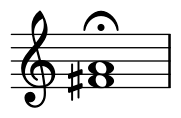According to the same page, a busy signal is a combination of 480 Hz and 620 Hz with pulses of 1/2 second.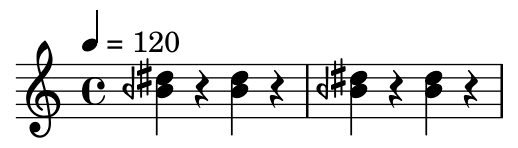Note that the bottom note is an B half flat, i.e. midway between a B and a B flat, denoted by the backward flat sign. The previous post on DTMF tones also used quarter tone notation because the frequencies don’t align well with a conventional chromatic scale. The frequencies were chosen to be easy to demodulate rather than to be musically in tune.

## Audio files

Here are audio files corresponding to the notation above.

## Lilypond code for music

Here is the Lilypond code that was used to make the images above.

    \begin{lilypond}
\new Staff \with { \omit TimeSignature} {
\relative c'{ 1 \fermata | }
}
\new Staff {
\tempo 4 = 120
\relative c''{
<beh dis> r4 <beh dis> r4 | <beh dis> r4 <beh dis> r4 |
}
}
\end{lilypond}


## Related posts

 Dual-tone multi-frequency signaling, trademarked as Touch-Tone

# Phone tones in musical notation

The sounds produced by a telephone keypad are a combination of two tones: one for the column and one for the row. This system is known as DTMF (dual tone multiple frequency).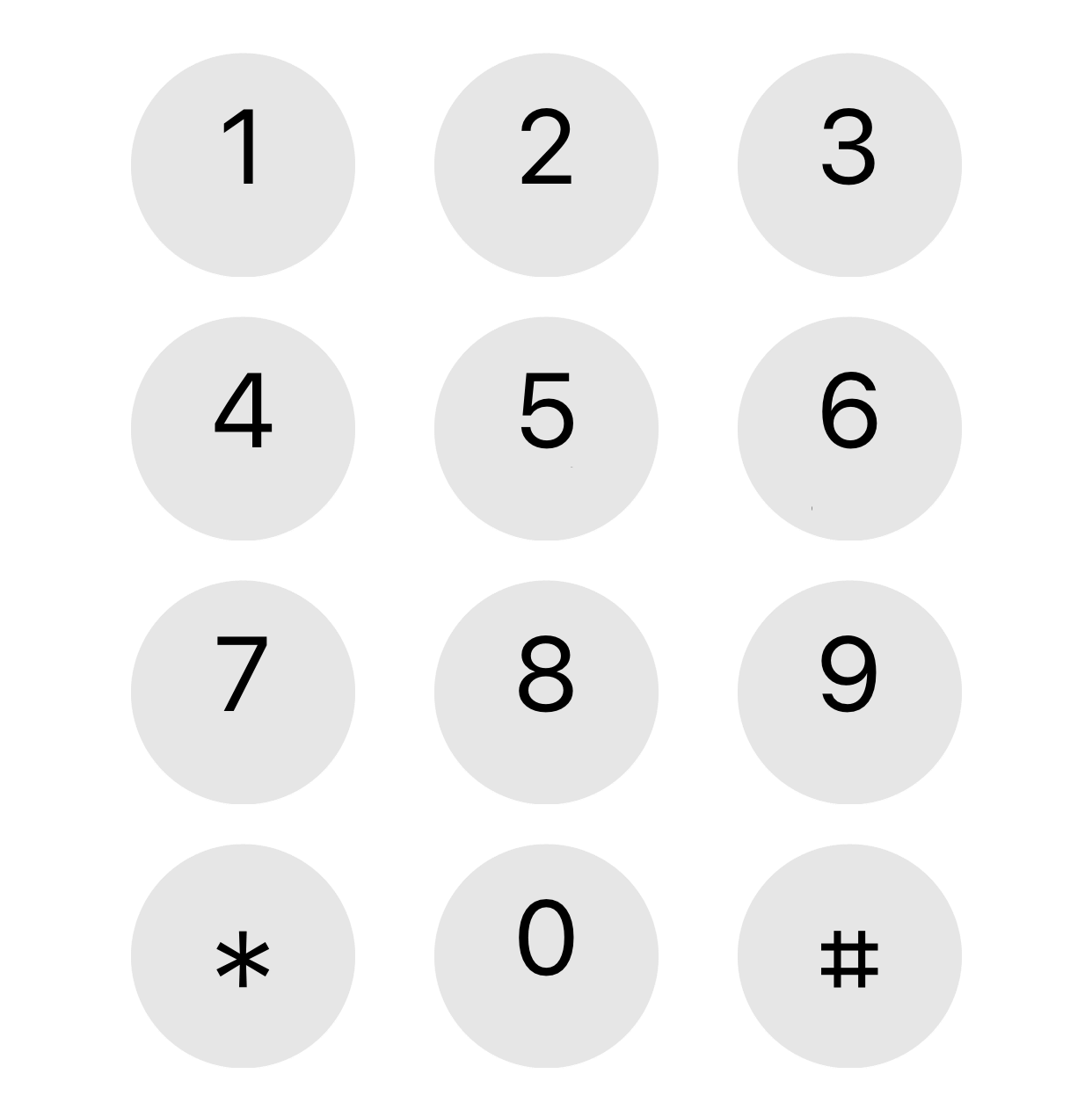I’ve long wondered what these tones would be in musical terms and I finally went to the effort to figure it out when I ran across DTMF in .

The three column frequencies are 1209, 1336, and 1477 Hz. These do not correspond exactly to standard musical pitches. The first frequency, 1209 Hz, is exactly between a D and a D#, two octaves above middle C. The second frequency, 1336 Hz, is 23 cents  higher than an E. The third frequency, 1477 Hz, lands on an F#.

In approximate musical notation, these pitches are two octaves above the ones written below.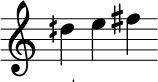Notice that the symbol in front of the D is a half sharp, one half of the symbol in front of the F.

Similarly, the four row frequencies, starting from the top, are 697, 770, 852, and 941 Hz. In musical terms, these notes are F, G (31 cents flat), A (54 cents flat), and B flat (16 cents sharp).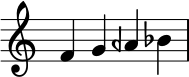The backward flat symbol in front of the A is a half flat. As with the column frequencies, the row frequencies are two octaves higher than written.

These tones are deliberately not in traditional harmony because harmonic notes (in the musical sense) are harmonically related (in the Fourier analysis sense). The phone company wants tones that are easy to pull apart analytically.

Finally, here are the chords that correspond to each button on the phone keypad.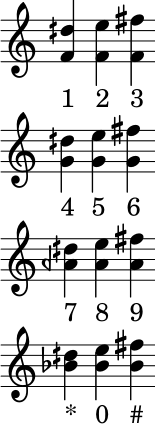Update: Dial tone and busy signal

## Related posts

 Electric Circuits by Nilsson and Riedel, 10th edition, page 548.
 A cent is 1/100 of a semitone.

# Frequency shift keying (FSK) spectrum

This post will look encoding digital data as an analog signal using frequency shift keying (FSK), first directly and then with windowing. We’ll look at the spectrum of the encoded signal and show that basic FSK uses much less bandwidth than direct encoding, but more bandwidth than FSK with windowing.

## Square waves

The most natural way to encode binary data as an analog signal would be represent 0s and 1s by a sequence of pulses that take on the values 0 and 1.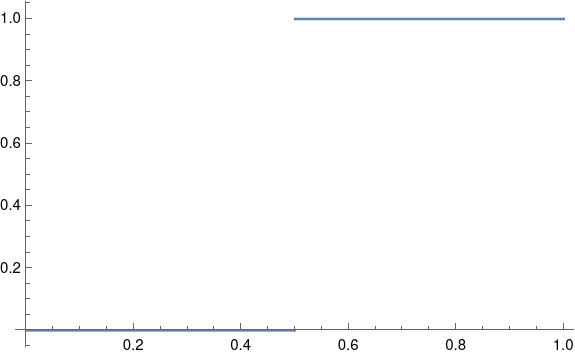A problem with this approach is that it would require a lot of bandwidth.

In theory a square wave has infinite bandwidth: its Fourier series has an infinite number of non-zero coefficients. In practice, the bandwidth of a signal is determined by how many Fourier coefficients it has above some threshold. The threshold would depend on context, but let’s say we ignore Fourier components with amplitude smaller than 0.001.

As I wrote about here, the nth Fourier sine series coefficients for a square wave is equal to 4/nπ for odd n. This means we would need on the order of 1,000 terms before the coefficients drop below our threshold.

## Frequency shift keying

The rate of convergence of the Fourier series for a function f depends on the smoothness of f. Discontinuities, like the jump in a square wave, correspond to slow convergence, i.e. high bandwidth. We can save bandwidth by encoding our data with smoother functions.

So instead of jumping from 0 to 1, we’ll encode a 0 as a signal of one frequency and a 1 as a signal with another frequency. By changing the frequency after some whole number of periods, the resulting function will be continuous, and so will have smaller bandwidth.

Suppose we have a one second signal f(t) that is made of half a second of a 4 Hz signal and half a second of a 6 Hz signal, possibly encoding a 0 followed by a 1.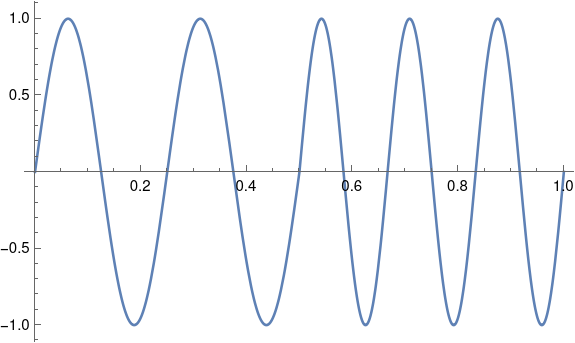What would the Fourier coefficients look like? If we just had a 4 Hz sine wave, the Fourier series would have only one component: the signal itself at 4 Hz. If we just had a 6 Hz sine wave, the only Fourier component would again be the signal itself. There would be no sine components at other frequencies, and no cosine components.

But our signal patched together by concatenating 4 Hz and 6 Hz signals has non-zero cosine terms for every odd n, and these coefficients decay like O(1/n²).

Our Fourier series is

f(t) = 0.25 sin 8πt + 0.25 sin 12πt + 0.0303 cos 2πt + 0.1112 cos 6πt – 0.3151 cos 12πt + 0.1083 cos 10πt + …

We need to go out to 141 Hz before the coefficients drop below 0.001. That’s a lot of coefficients, but it’s an order of magnitude fewer coefficients than we’d need for a square wave.

## Pulse shaping

Although our function f is continuous, it is not differentiable. The left-hand derivative at 1/2 is 8π and the right-hand derivative is 12π. If we could replace f with a related function that is differentiable at 1/2, presumably the signal would require less bandwidth.

We could do this by multiplying both halves of our signal by a windowing function. This is called pulse shaping because instead of a simple sine wave, we change the shape of the wave, tapering it at the ends.

Let’s using a cosine window because that’ll be easy; in practice you’d probably use a different window .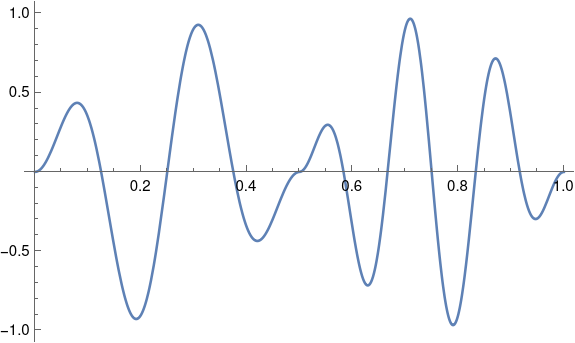Now our function is differentiable at 1/2, and its Fourier series converges more quickly. Now we can disregard components above 40 Hz. With a smoother windowing function the windowed function would have more derivatives and we could disregard more of the high frequencies.

## Related posts

 This kind of window is called a cosine window because you multiply your signal by one lobe of a cosine function, with the peak in the middle of the signal. Since we’re doing this over [0. 1/2] and again over [1/2, 1], we’re actually multiplying by |sin 2πt|.

# Aliasing in a nutshell

Suppose you have a sine wave with frequency f0 Hz.We’re going to discretize this signal by sampling it fs times per second. That is, we’re going to evaluate S at integer multiples ofThe result is the sequencewhere n runs through the integers.

Next, let k be an integer and consider the sine wave(Foreshadowing: A is for “alias.”)

Now let’s sample A at the same frequency as S, i.e. fs Hz. This gives us the sequenceA short derivation showswhich is exactly what we got from sampling S.

To recap, sampling a signal of f0 Hz at a rate of fs Hz produces the same samples as sampling a signal of f0 + kfs Hz at the same rate for any integer k.

So, for example, if we’re sampling signals at 1000 samples per second, then we’ll get the same samples whether we’re sampling a signal of 440 Hz or 1440 Hz or 2440 Hz etc.

Periodic sampling cannot distinguish frequency components that differ by an integer multiple of the sampling frequency.

If a signal has components at 440 Hz and at 1440 Hz, and we sample at 1000 Hz, all the information from the higher frequency component is aliased, added to the samples of the 440 Hz component.

If a signal contains frequencies between –B and B, you can avoid aliasing by sampling at a rate higher than 2B. In practice this may mean that your signal has frequency components outside the interval [-B, B] but these components are small enough to ignore.

A good rule of thumb is to sample at a frequency of at least 2.5B and not just the theoretical minimum of 2B. For more on this, see The Engineer’s Nyquist frequency.

# FM signal approximation

FM radio transmits a signal by perturbing (modulating) the frequency of a carrier wave. If the carrier has frequency ω and the signal has frequency q, then the FM signal is

cos(ωt + β cos(qt)).

To understand the modulated signal, it’s useful to write it as a sum of simple sines and cosines with no modulation. I wrote about how to do this exactly using Bessel functions. Today I’ll write about an approximation that’s easier to understand and work with, assuming the modulation index β is small.

Here’s the approximation:

cos(ωt + β cos(qt)) ≈ cos ωt + ½ β ( sin (ω + q)t + sin (ω – q)t ).

This says that to a good approximation, the modulation term adds two sine waves to the carrier, one that adds the signal frequency to the carrier frequency and one that subtracts it.

To establish the approximation and see how the error depends on β, subtract the right side from the left and expand as a Taylor series in β. The first non-zero term in the series is

-½ cos(qt)² cos(ωt) β²

and so if β is small, the approximation error is very small. For example, if β = 0.1, then the approximation error is on the order of 0.005.

As an example, let ω = 10, q = 2, and β = 0.1. Then

cos(10t + 0.1 cos 2t) ≈ cos 10t + 0.05 ( sin 12t + sin 8t )

and the approximation error is plotted below.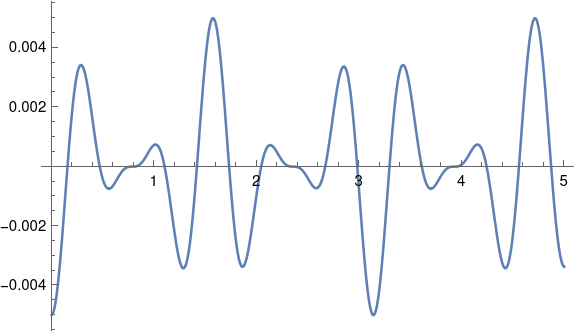As predicted, the amplitude of the error is around 0.005, while the amplitude of the FM signal is 1.

# Identifying someone from their heart beatHow feasible would it be to identify someone based from electrocardiogram (EKG, ECG) data? (Apparently the abbreviation “EKG” is more common in America and “ECG” is more common in the UK.)

Electrocardiograms are unique, but unique doesn’t necessarily mean identifiable. Unique data isn’t identifiable without some way to map it to identities. If you shuffle a deck of cards, you will probably produce an arrangement that has never occurred before. But without some sort of registry mapping card deck orders to their shufflers, there’s no chance of identification. (For identification, you’re better off dusting the cards for fingerprints, because there are registries of fingerprints.)

According to one survey , researchers have tried a wide variety of methods for identifying people from electrocardiograms. They’ve used time-domain features such as peak amplitudes, slopes, variances, etc., as well as a variety of frequency-domain (DFT) features. It seems that all these methods work moderately well, but none are great, and there’s no consensus regarding which approach is best.

If you have two EKGs on someone, how readily can you tell that they belong to the same person? The answer depends on the size of the set of EKGs you’re comparing it to. The studies surveyed in  do some sort of similarity search, comparing a single EKG to tens of candidates. The methods surveyed had an overall success rate of around 95%. But these studies were based on small populations; at least at the time of publication no one had looked at matching an single EKG against thousands of possible matches.

In short, an electrocardiogram can identify someone with high probability once you know that they belong to a relatively small set of people for which you have electrocardiograms.

## More identification posts

 Antonio Fratini et al. Individual identification via electrocardiogram analysis. Biomed Eng Online. 2015; 14: 78. doi 10.1186/s12938-015-0072-y